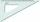# Right triangle - examples - page 22

1. Is right triangleDecide if the triangle XYZ is rectangular: x = 4 m, y = 6 m, z = 4 m
2. RulerHow far from Peter stands 2m hight John? Petr is looking to John over ruler that keeps at arm's distant 60 cm from the eye and on the ruler John measured the height of 15 mm.
3. Right triangle ABCCalculate the perimeter and area of a right triangle ABC, if you know the length of legs 4 cm 5.5 cm and 6.8 cm is hypotenuse.
4. TreeBetween points A and B is 50m. From A we see a tree at an angle 18°. From point B we see the tree in three times bigger angle. How tall is a tree?
5. Land - isosceles trapezoidCalculate the content and perimeter of the building plot in the form of an isosceles trapezoid with bases 120m, 95m and height 50m.
6. Tree shadow 3A 2-meter rod casts a shadow 3.2 m long. How high is a tree with a shadow of 14.4 m ?
7. Chord circleThe circle to the (S, r = 8 cm) are different points A, B connected segment /AB/ = 12 cm. AB mark the middle of S'. Calculate |SS'|. Make the sketch.
8. HypotenuseCalculate the length of the hypotenuse of a right triangle with a catheti 71 cm and 49 cm long.
9. Cube in sphereThe sphere is inscribed cube with edge 8 cm. Find the radius of the sphere.
10. Body diagonalFind the cube surface if its body diagonal has a size of 6 cm.
11. Regular quadrilateral pyramidFind the volume and surface of a regular quadrilateral pyramid if the bottom edge is 45 cm long and the pyramid height is 7 cm.
12. The ladderThe ladder is 10 m long The ladder is 8 m high How many meters is the distant heel from the wall?
13. ForcesForces with magnitudes F1 = 42N and F2 = 35N act at a common point and make an angle of 77°12'. How big is their resultant?
14. MidpointsTriangle ABC with sides a = 5 cm, b = 3 cm, c = 40mm has a midpoint of K, L, M. How many centimeters is long perimeter of parallelogram KBLM?
15. KiteJohn a kite, which is diamond shaped. Its diagonals are 60 cm long and 90 cm long. Calculate: a) the diamond side b) how much paper John needs to make a kite if he needs paper on both sides and needs 5% of the paper for bending.
16. Wall heightCalculate the surface and volume of a regular quadrangular pyramid if side a = 6 cm and wall height v = 0.8dm.
17. ConeThe rotating cone volume is 9.42 cm3, with a height 10 cm. What angle is between the side of the cone and its base?
18. The ditchDitch with cross section of an isosceles trapezoid with bases 2m 6m are deep 1.5m. How long is the slope of the ditch?
19. Circular ringSquare with area 16 centimeters square are inscribed circle k1 and described circle k2. Calculate the area of circular ring, which circles k1, k2 form.
20. Equilateral triangleThe equilateral triangle has a 23 cm long side. Calculate its content area.

Do you have an interesting mathematical example that you can't solve it? Enter it, and we can try to solve it.

To this e-mail address, we will reply solution; solved examples are also published here. Please enter e-mail correctly and check whether you don't have a full mailbox.

See also our right triangle calculator.